# How to Calculate and Solve for Superelevation, Guage of Track, Velocity and Radius of a Body in Circular Path Motion | The Calculator Encyclopedia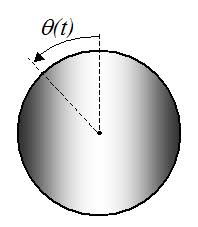The image above represents a body in superelevation.

To compute for the Superelevation, four essential parameters are needed and these parameters are Gauge of the track (G), velocity of the body (v), radius of the curve (r) and acceleration due to gravity (g).

The formula for calculating the circular path motion:

S = Gv² / gr

Where:
S = Superelevation
G = Gauge of the track
v = velocity of the body
r = radius of the curve
g = acceleration due to gravity

Let’s solve an example;
Find the circular path motion when Gauge of the track is 14, velocity of the track is 47, radius of the curve is 21 and acceleration due to gravity is 9.8.

This implies that;
G = Gauge of the track = 14
v = velocity of the body = 47
r = radius of the curve = 21
g = acceleration due to gravity = 9.8

S = Gv² / gr
S = 14 x 47² / 9.8 x 21
S = 14 x 2209 / 205.8
S = 30926 / 205.8
S = 150.27

Therefore, the superelevation is 150.27 m.

Calculating the Gauge of the track when Superelevation, Velocity of the body, Radius of the curve and Acceleration due to gravity.

G = Sgr / v2

Where;
G = Gauge of the track
S = Superelevation
v = velocity of the body
r = radius of the curve
g = acceleration due to gravity

Let’s solve an example;
With a superelevation of 180, velocity of the body is 32, radius of the curve is 12 and acceleration due to gravity as 9.8. Find the gauge of the track?

This implies that;
S = Superelevation = 180
v = velocity of the body = 32
r = radius of the curve = 12
g = acceleration due to gravity = 9.8

G = Sgr / v2
G = 180 x 12 x 9.8 / 322
G = 21168 / 1024
G = 20.67

Therefore, the gauge of the track is 20.67.

Calculating the Velocity of the body when Superelevation, Gauge of the track, Radius of the curve and Acceleration due to gravity.

v = √Sgr / G

Where;
v = velocity of the body
S = Superelevation
G = Gauge of the track
r = radius of the curve
g = acceleration due to gravity

Let’s solve an example;
With a superelevation of 120, gauge of the track is 28, radius of the curve is 7 and acceleration due to gravity as 9.8. Find the velocity of the body?

This implies that;
S = Superelevation = 120
G = gauge of the track = 28
r = radius of the curve = 7
g = acceleration due to gravity = 9.8

v = √Sgr / G
v = √120 x 9.8 x 7 / 28
v = √8232 / 28
v = √294
v = 17.146

Therefore, the velocity of the body is 17.146.

Calculating the Radius of the curve when Superelevation, Gauge of the track, Velocity of the body and Acceleration due to gravity.

r = Gv2 / Sg

Where;
r = radius of the curve
v = velocity of the body
S = Superelevation
G = Gauge of the track
g = acceleration due to gravity

Let’s solve an example;
With a superelevation of 170, gauge of the track is 18, velocity of the body is 9 and acceleration due to gravity as 9.8. Find the radius of the curve?

This implies that;
S = Superelevation = 170
G = gauge of the track = 18
v = velocity of the body = 9
g = acceleration due to gravity = 9.8

r = Gv2 / Sg
r = 18 x 92 / 170 x 9.8
r = 1458 / 1666
r = 0.875

Therefore, the radius of the curve is 0.875.

Nickzom Calculator – The Calculator Encyclopedia is capable of calculating the superelevation.

To get the answer and workings of the superelevation using the Nickzom Calculator – The Calculator Encyclopedia. First, you need to obtain the app.

You can get this app via any of these means:

To get access to the professional version via web, you need to register and subscribe for NGN 1,500 per annum to have utter access to all functionalities.
You can also try the demo version via https://www.nickzom.org/calculator

Once, you have obtained the calculator encyclopedia app, proceed to the Calculator Map, then click on Mechanics under Engineering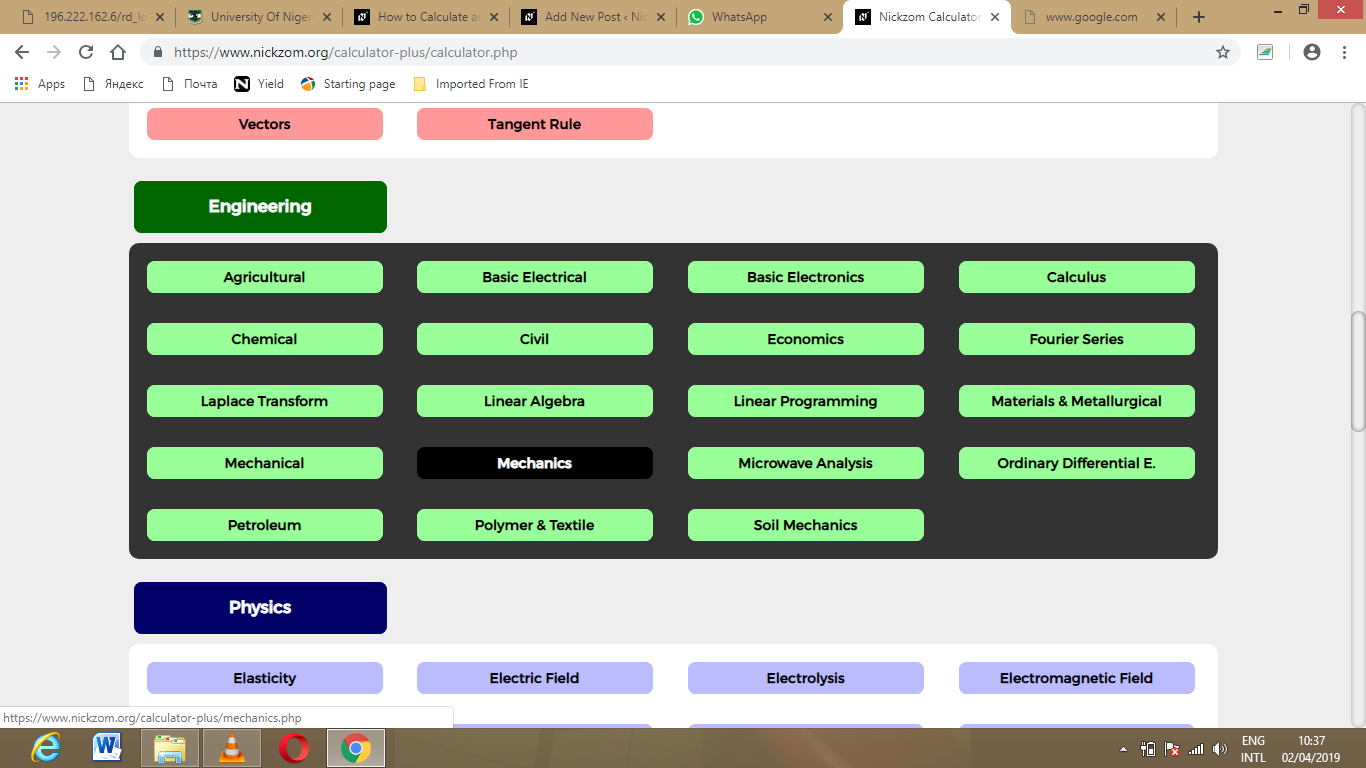Now, Click on Motion of Circular Path under Mechanics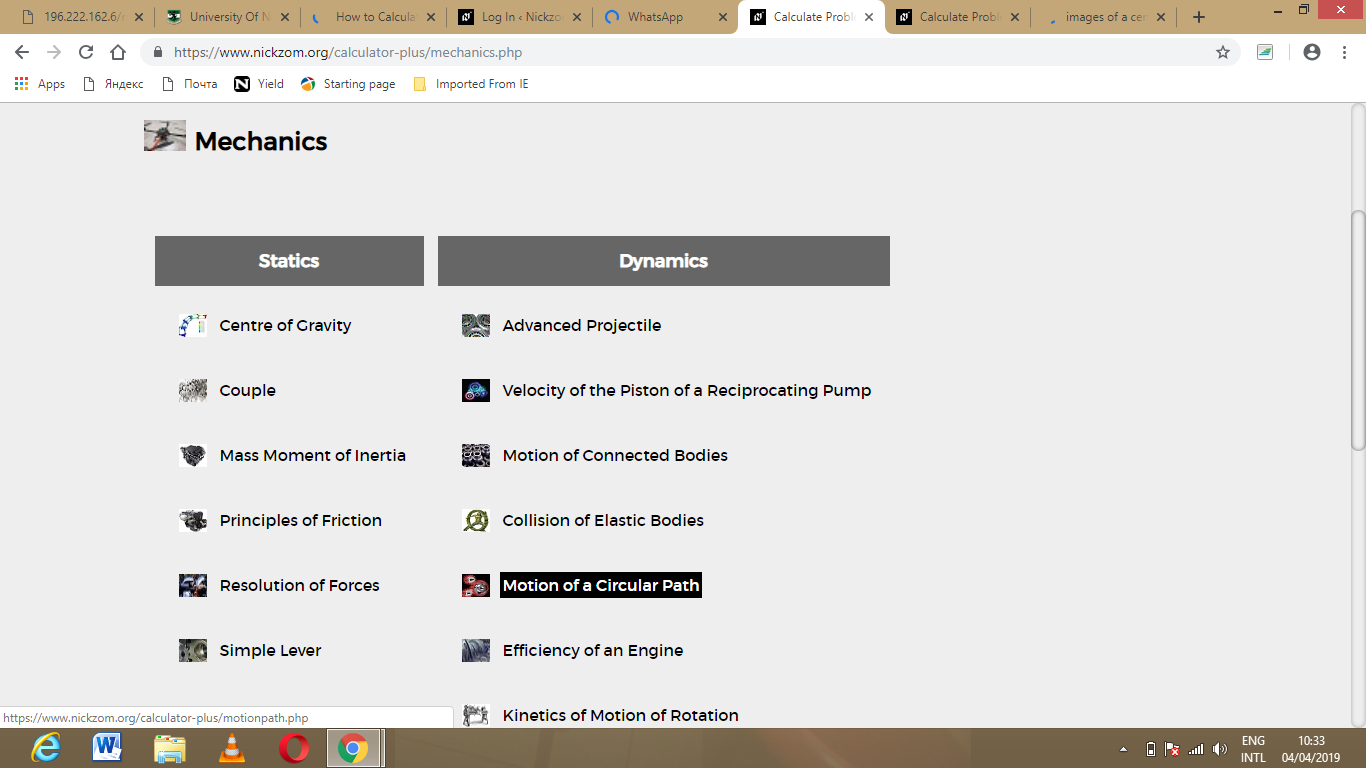Click on Superelevation under Motion of Circular PathThe screenshot below displays the page or activity to enter your value, to get the answer for the superelevation according to the respective parameter which are the Gauge of the track (G), velocity of the body (v), radius of the curve (r) and acceleration due to gravity (g).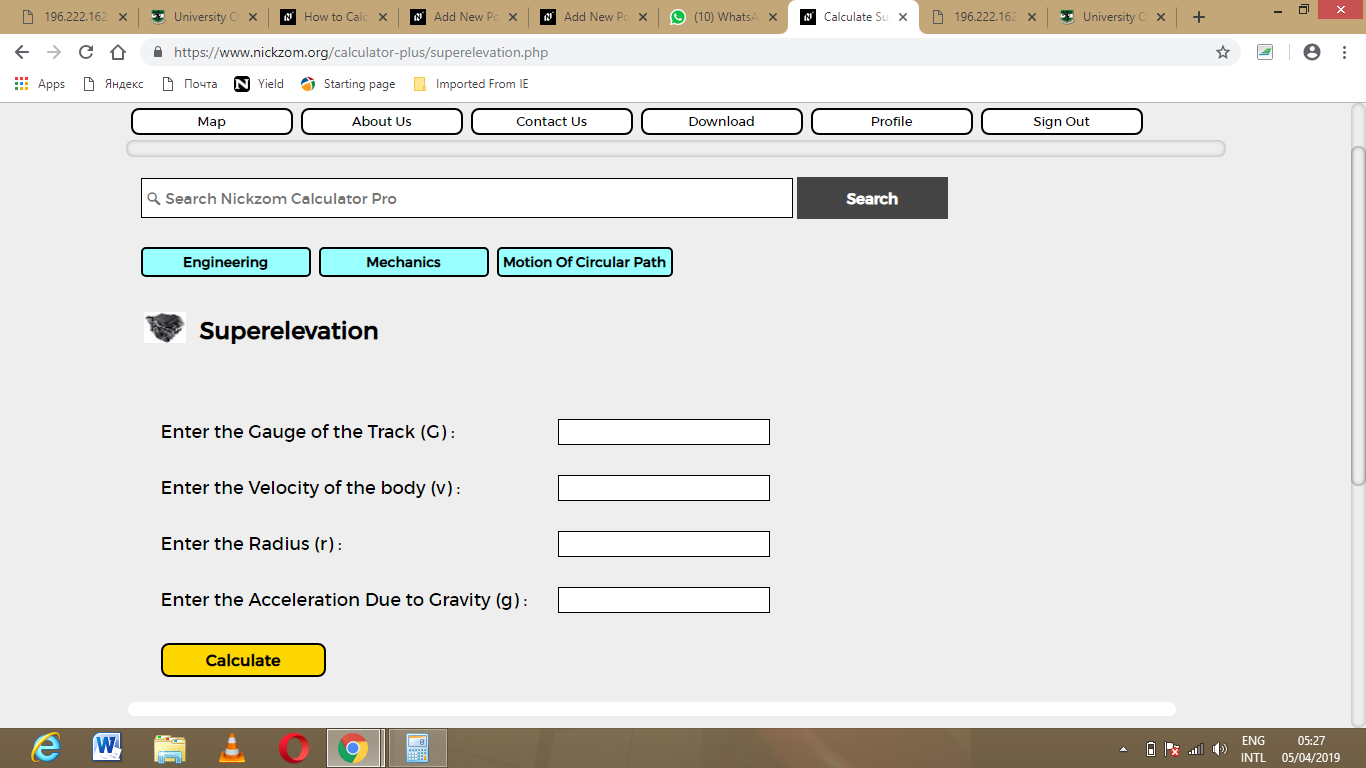Now, enter the value appropriately and accordingly for the parameter as required by the Gauge of the track (G) is 14, velocity of the body (v) is 47, radius of the curve (r) is 21 and acceleration due to gravity (g) is 9.8.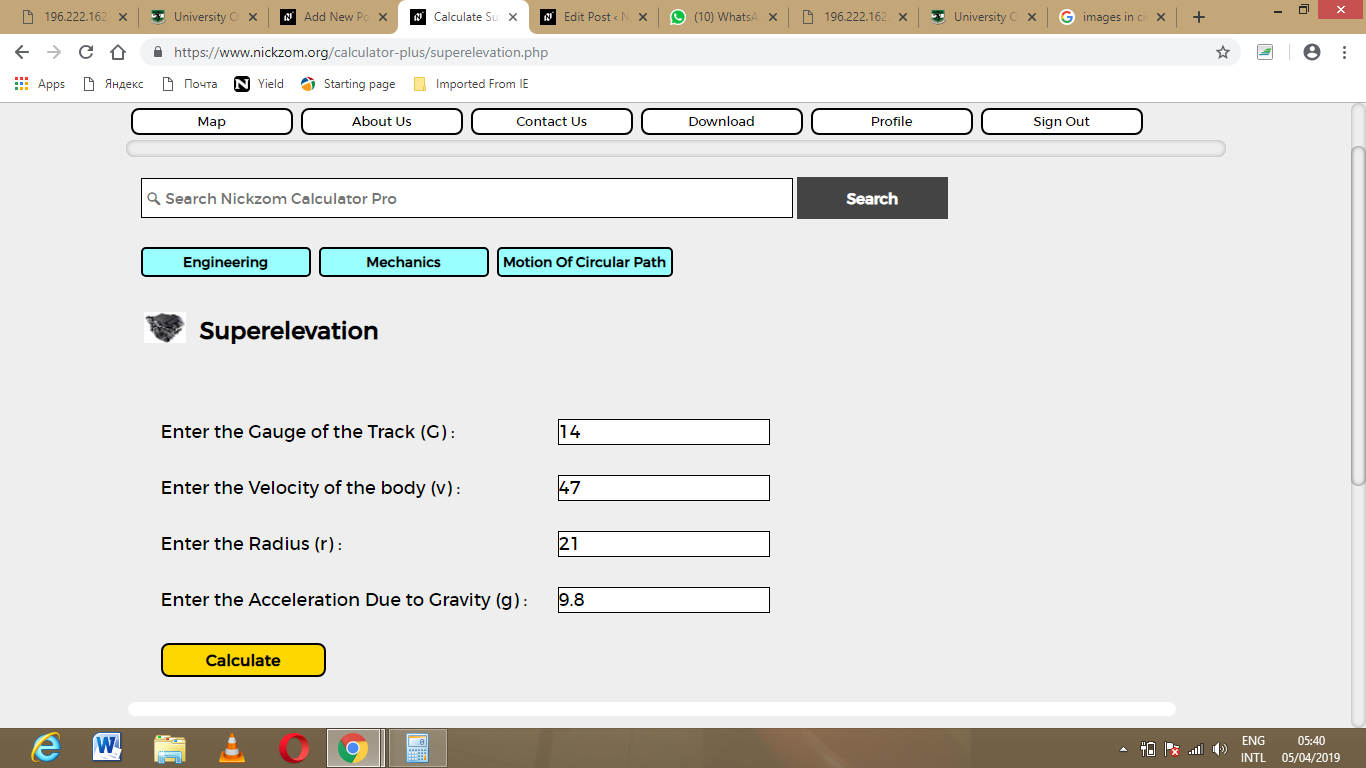Finally, Click on Calculate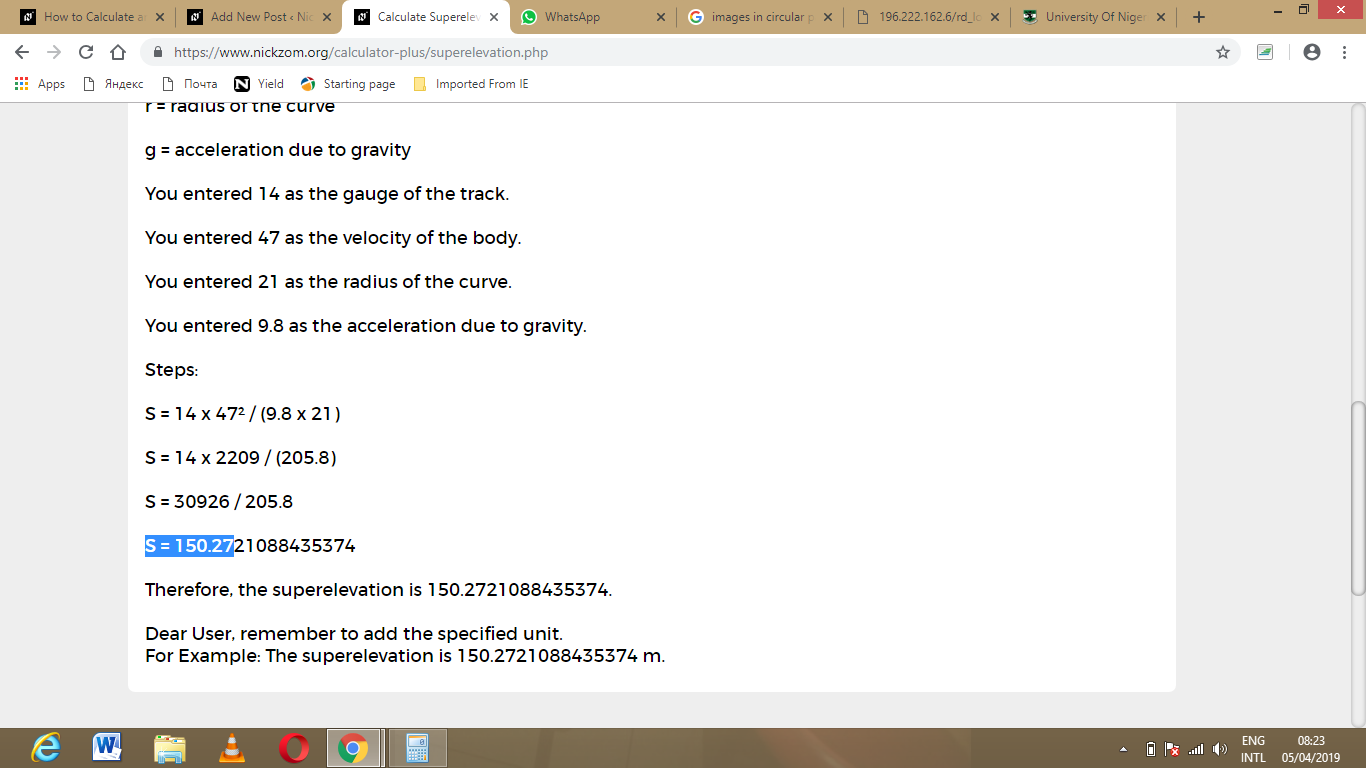As you can see from the screenshot above, Nickzom Calculator – The Calculator Encyclopedia solves for the superelevation and presents the formula, workings and steps too.